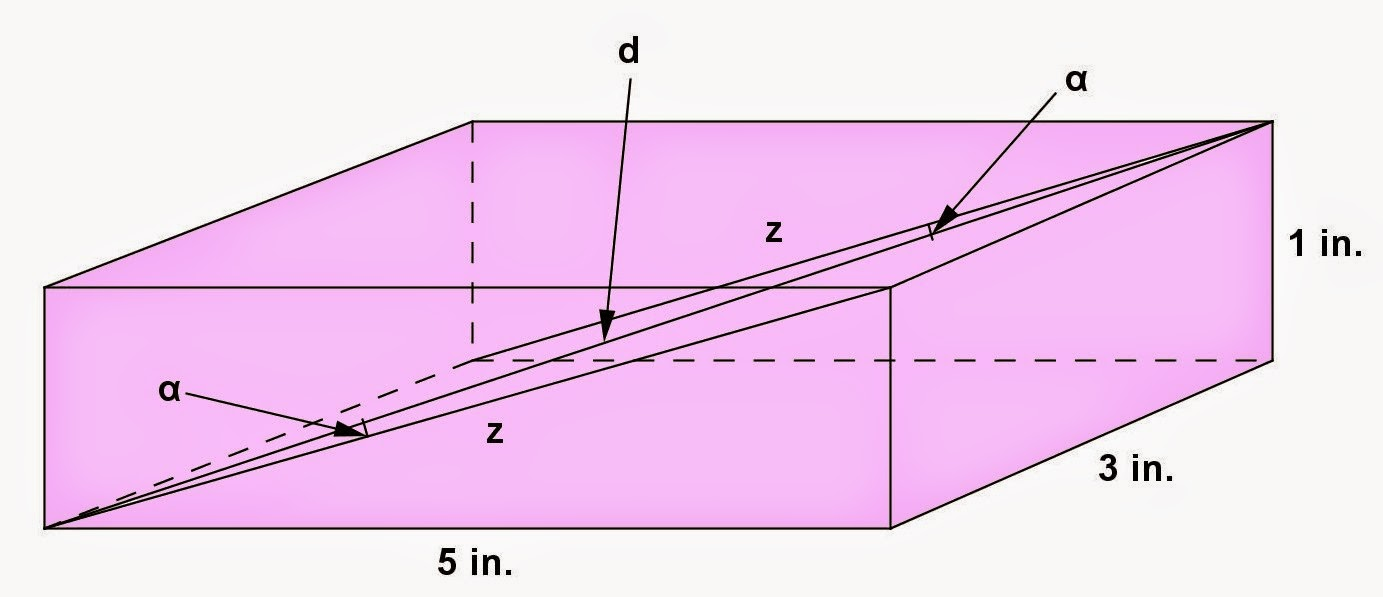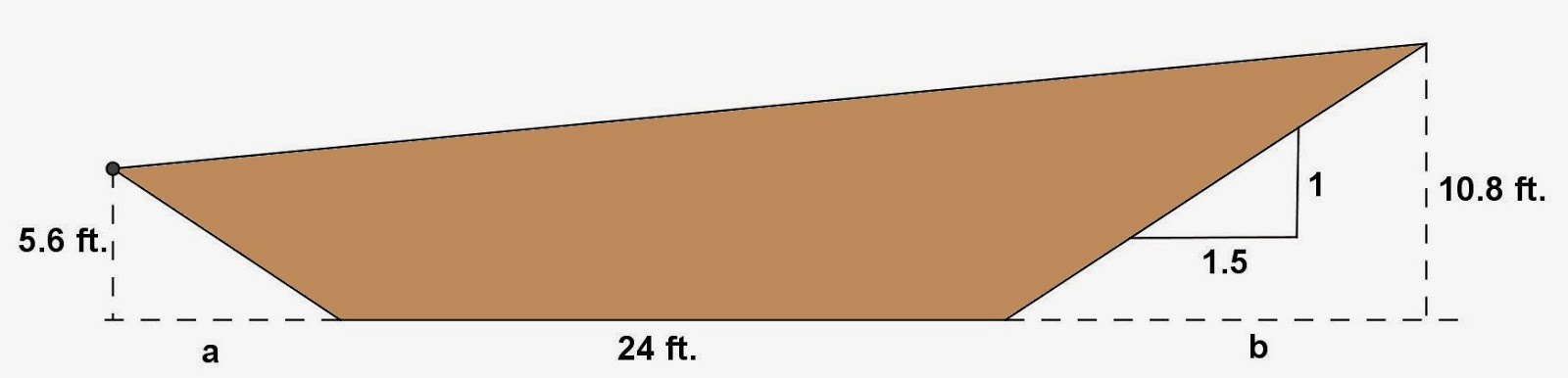## Saturday, February 28, 2015

### Rectangular Parallelepiped Problems, 17

Category: Solid Geometry

"Published in Newark, California, USA"

Find the angles that the diagonal of a rectangular parallelepiped 1 in. by 3 in. by 5 in. make with the faces.

Solution:

To illustrate the problem, it is better to draw the figure as followsPhoto by Math Principles in Everyday Life

Next, we need to draw a diagonal line at the top and bottom of the rectangular parallelepiped as well as its diagonal and angles as followsPhoto by Math Principles in Everyday Life

By using Pythagorean Theorem, the length of d is

Therefore, the value of an angle is

or

Next, we need to draw a diagonal line at the left and right of the rectangular parallelepiped as well as its diagonal and angles as followsPhoto by Math Principles in Everyday Life

Therefore, the value of an angle is

or

Next, we need to draw a diagonal line at the front and back of the rectangular parallelepiped as well as its diagonal and angles as followsPhoto by Math Principles in Everyday Life

Therefore, the value of an angle is

or

## Friday, February 27, 2015

### Trapezoid Prism Problems, 8

Category: Solid Geometry

"Published in Newark, California, USA"

The figure shows a right section of a railroad cut in a hillside. If the sides rise 1 unit vertically to a horizontal distance of 1.5 units, and if the length of the cut is 100 ft., find the volume of earth removed.Photo by Math Principles in Everyday Life

Solution:

The cross section of a railroad cut in a hillside is a general quadrilateral. If you draw vertical lines from the top ends of a general quadrilateral to the bottom horizontal line that contains one side, then it becomes a trapezoid as followsPhoto by Math Principles in Everyday Life

By similar triangles, we can solve for the values of a and b as follows

The area of a trapezoid is

The area of small triangle is

The area of big triangle is

Hence, the area of the base which is the cross section of a railroad cut in a hillside is

Therefore, the volume of earth removed which is the volume of a prism is